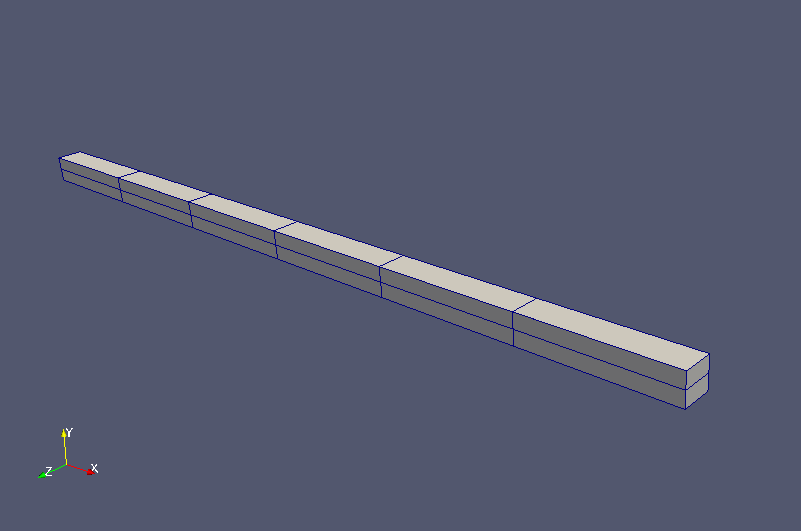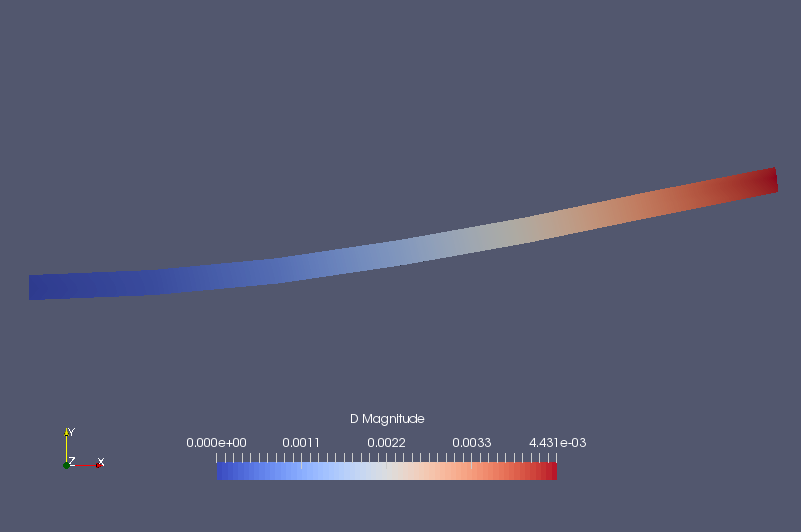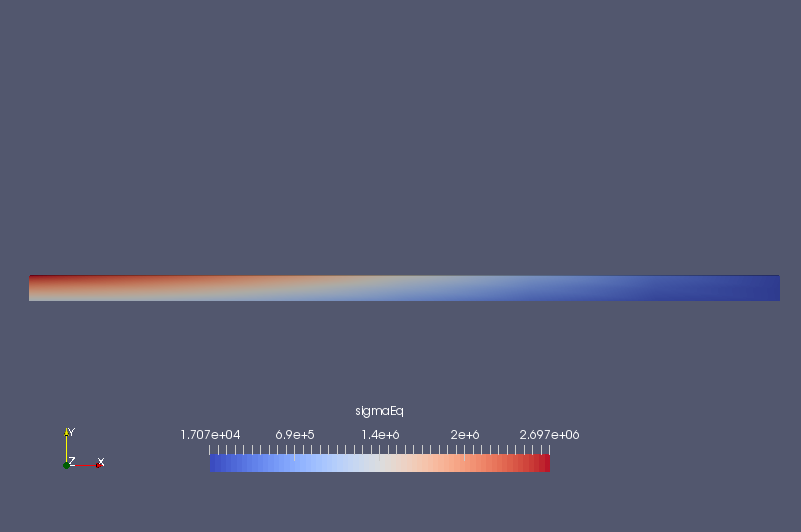﻿ Stress analysis of a beam - XSim

# Stress analysis of a beam

OpenFOAM 4.x

## Summary

This is a stress analysis of a loaded beam in a balanced state. We will fix the region fixedEnd on the left end of the beam, and load (10000, 0, 0) Pa to the region right on the right end of the beam. The calculation is performed as a two-dimensional problem with a single mesh in the Z direction.Model geometry

The meshes are as follows, and the number of mesh is 12.Meshes

The calculation result is as follows.Displacement (D, multiplied by 1000 times and reflected in the shape)von Mises stress (sigmaEq)